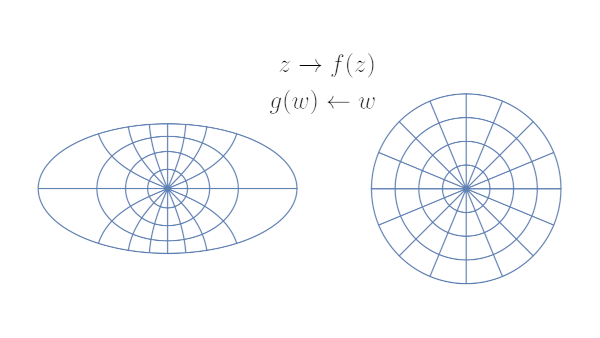# Conformal map of ellipse interior to a disk

This post will present the conformal map between the interior of an ellipse and the unit disk.Given an ellipse centered at the origin with semi-major axis a and semi-minor axis b. Will will assume without loss of generality that a² – b² = 1 and so the foci are at ±1.

Hermann Schwarz published the conformal map from the ellipse to the unit disk in 1869 [1, 2].

The map is given bywhere sn is the Jacobi elliptic function with parameter k². The constants k and K are given bywhere θ2 and θ3 are theta constants, the value so the theta functions θ2(z, q) and θ3(z, q) at z = 1.

Conformal maps to the unit disk are unique up to rotation. The map above is the unique conformal map preserving orientation:## Inverse map

The inverse of this map is given byThe inverse of the sn function with parameter m can be written in terms of elliptic integrals.where F is the incomplete elliptic integral of the first kind and m is the parameter of sn and the parameter of F.

## Plot

I wanted to illustrate the conformal map using an ellipse with aspect ratio 1/2. To satisfy a² – b² = 1, I set a = 2/√3 and b = 1/√3. The plot at the top of the post was made using Mathematica.

## Related posts

 H. A. Schwarz, Über eigige Abbildungsaufgaben, Journal für di reine und angew. Matheamatik, vol 70 (1869), pp 105–120

 Gabor Szegö. Conformal Mapping of the Interior of an Ellipse onto a Circle. The American Mathematical Monthly, 1950, Vol. 57, No. 7, pp. 474–478